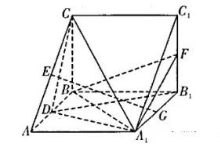(1)求证: $G E / /$ 平面 $B C C_1 B_1$;
(2) 若二面角 $B_1-A_1 D-C$ 的余弦值为 $\frac{\sqrt{21}}{21}$, 且 $A B=2$, 求多面体 $A B C A_1 F C_1$ 的体积.①点击 首页查看更多试卷和试题 , 点击查看 本题所在试卷